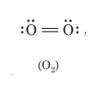# Calculate the bond order of O2.

All the molecule posses bond order. The bond order varies from one molecule to another. Oxygen is a diatomic molecule. Let us first know what do you mean by bond order.

## Bond order

The bond order may be defined as half the difference between the number of electrons in bonding molecular orbitals (Nonbonding) and the number of electrons in the antibonding molecular orbital.

### The bond order of O2

The bond order is the number of bonds present between two atoms in a molecule or ion. Various molecular properties can be understood by this concept such as variation in bond length and bond enthalpies.For oxygen, the bond order is 2.

## Bonds in oxygen molecule

There are two covalent bonds present between the two bonded atoms. Out of these two bonds,

• One is always sigma bond
• The second is pi bond.